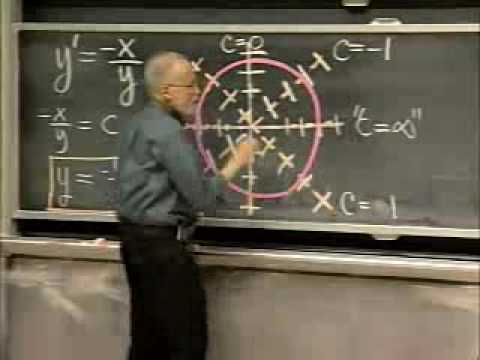MIT OpenCourseWare

# Differential Equations

this course is a study of Ordinary Differential Equations (ODEs), including modeling physical systems. Differential Equations are the language in which the laws of nature are expressed. Understanding properties of solutions of differential equations is fundamental to much of contemporary science and engineering. Ordinary differential equations (ODEs) deal with functions of one variable, which can often be thought of as time. Topics covered in this course include: Solution of first-order ODEs by analytical, graphical and numerical methods; Linear ODEs; Undetermined coefficients and variation of parameters; Sinusoidal and exponential signals; Complex numbers and exponentials; Fourier series, periodic solutions; Delta functions, convolution, and Laplace transform methods; Matrix and first order linear systems; and Nonlinear autonomous systems. (from ocw.mit.edu )

Instructor

Mathematics
2008

#### Lecture 01 - The Geometrical View of y'= f(x,y): Direction Fields, Integral Curves